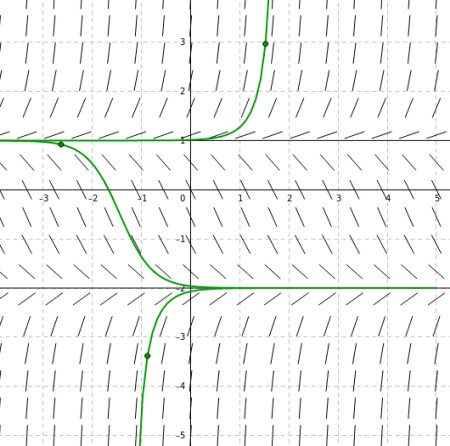# For the differential equation y'=(y+2)(y-1), draw the y'=0 curves, determine the direction of...

## Question:

For the differential equation {eq}y'=(y+2)(y-1) {/eq}, draw the {eq}y'=0 {/eq} curves, determine the direction of motion in each region, and draw representative solutions.

## Phase Plot of First Order Differential Equations

The phase plot of a first order differential equation {eq}\displaystyle y'=f(x,y) {/eq} is a 2D plot of slopes of the general solution of the equation.

Taking {eq}\displaystyle y'=0\iff f(x,y)=0, {/eq} we will obtain the solution curve has horizontal tangent lines.

Next, we will plot tangents corresponding to positive and negative slope.

Depending on the initial condition, we will be able to sketch the solution (curve) of the differential equation.

To determine the direction of motion in each region of the differential equation {eq}\displaystyle y'=(y+2)(y-1) {/eq}

which is an autonomous equation, because the independent variable is not explicitly shown,

we will first find the horizontal tangent lines.

{eq}\displaystyle y'=0\iff (y+2)(y-1)=0\implies \text{ the lines }y=-2, y=1 {/eq} are the horizontal tangent lines to the solution.

Below and above the two lines, the function {eq}\displaystyle (y+2)(y-1)>0 {/eq} so, the slopes are positive, the solution moves toward {eq}\displaystyle y=-2, {/eq} and away from {eq}\displaystyle y=1. {/eq}

Between {eq}\displaystyle y=-2 \text{ and } y=1, {/eq} the slope is negative, {eq}\displaystyle (y+2)(y-1)<0, {/eq} so the solution moves toward -2 and away from 1.

The slopes are shown in the picture below as short black segments and three possible solutions, are shown with green solid line, depending on the initial condition.

For example, if the initial condition is {eq}\displaystyle y(0)<-2 {/eq} the curve is shown below the line {eq}\displaystyle y=-2. {/eq}

The curves {eq}\displaystyle y'=0 \text{ which are } y=-2, y=1 {/eq} are shown with the solid black lines.